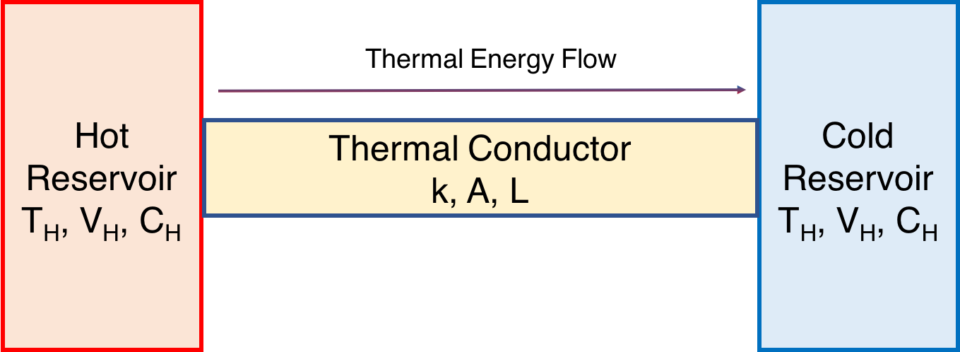# First Law of Thermodynamics

#### By Mark Ciotola

First published on May 16, 2019. Last updated on April 30, 2021.

## The Law

The First Law of Thermodynamics states that the total energy of an isolated system shall not change. In other words, the First Law of Thermodynamics requires that energy can neither be created nor destroyed. In other words, energy is conserved. This simply means that if heat flows from one object to another, the quantity of heat leaving the first object must equal the quantity of heat entering the second object.

## Discussion

The total energy shall neither increase more decrease. In physics, we say that the energy of an isolated system is conserved. (In physics, the term conserved has nothing to do with the environmental term of conservation).

What is an isolated system? Not surprisingly, a characteristic of isolated systems is that energy can neither enter or leave them. For example, a perfectly well-insulated container of hot water would be an isolated system. Although that particular hot water system is impossible in real life, it can be fairly well approximated using a reflective vacuum chamber, such as in an old-style coffee thermos. The entire Universe is considered to be an isolated system.

## Mixing example

A simple example that demonstrates the First Law is to mix a quantity of cool water with an equal quantity of hot water. If the water is kept in insulated containers before and after the mixing, then the temperature of the final mixture will be the mean of the temperatures of the original constituents (there may be a slight variation due to evaporation or escaped heat). In other words, the total amount of heat energy remained the same despite the mixing and temperature changes.

## Conduction example

Another simple example concerns a thermal conductor through which thermal energy flows from a warmer body of to a cooler body. The rate of thermal energy transfer Q is known as Newton’s Law of Cooling. We call the bodies reservoirs. The “hot” reservoir has a temperature of TH, and the “cold” reservoir has a temperature of TC. The conductor is of area (cross section) A, and of length L.Conductor bridging a thermal difference

The flow of thermal energy is:

$$\Large{\frac{dQ}{dt}}=kA\frac{T_H – T_C}{L}$$.

The quantity of heat energy lost by the warmer body is identical to the quantity of heat energy gained by the cooler body. This example can be easily replicated by using a U-shaped aluminum conductor to bridge two well-insulated cups of water of different temperatures.(The conductor should be appropriately insulated as well for best results).

Use the following simulation to try out Fourier’s Law. (The simulation may take a moment to load).

## Conversion of Form of Energy

The First Law allows energy to be transformed from one form into another, such as from potential to kinetic energy. Yet the total amount of energy must remain the same.

Consider the case of a swinging pendulum (in a vacuum). As the pendulum bob falls, it will speed up, and its kinetic energy shall consequently increase, while its potential energy (due to gravity) shall decrease. When the bob rises, its potential energy increases at the cost of its kinetic energy. In all cases, though, the total energy remains the same:

$$Total~Energy = Kinetic~Energy+Potential~Energy+Thermal~Energy$$.

Likewise, there is no change in the total energy of the system:

$$\Delta T + \Delta V + \Delta Q = 0$$,

where

$$\Delta T$$ is the change in kinetic energy, $$\Delta V$$ the change in potential energy, and $$\Delta T$$ the change in thermal energy.

What if we now have the pendulum operate in air, instead of a vacuum? The pendulum will gradually slow down due to air resistance. The total of potential + kinetic energy shall decrease! However, according to the First Law, the energy must go somewhere. It cannot merely disappear. As friction continues to operate and the pendulum continues to hit air molecules, the average (mean) velocity of the individual air molecules increases. So the air heats up a bit, and the pendulum’s energy is gradually transferred into heat energy. Eventually, the motion of the pendulum will stop, but energy in the air will have increased, as exhibited by an increased air temperature.

## Modern Physics Modification

Who has not heard of Albert Einstein’s famous equation $$E = mc^2$$? Energy can be changed into mass and vice-versus. So the First Law must be modified to take into account this mass-energy equivalence. This is the realm of nuclear reactors and nuclear bombs, and its effect is insignificant on most systems we encounter in our lives.

## Resources

• Paper concerning Fourier’s Law of Thermal Conduction
• Paper concerning Newton’s Law of Cooling and a simulation.
• Newton’s Law of Cooling simulation.
• Newton’s Law of Cooling program code.

## Notes & References

The phrase “conservation of energy” has a much different meaning than the common phrase “conserving energy”. The latter refers to consuming less of useful forms of energy such as coal or petroleum.

Such demonstration kits are commonly sold by science education equipment firms. If ice water is used, then energy due to the phase change of melting ice must also be accounted for.